Next: Genetic Algorithm [3* P] Up: MLB_Exercises_2010 Previous: Comparison of Optimization Algorithms

# Cart-Pole Controller Optimization [5 P]

Optimize the weights of a neural network that controls the horizontal forces applied to a cart in order to swing up a pole that is mounted on it into the upright position. Use an optimization algorithm of your choice (one of the four algorithms used in homework assignment 1). The state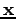of the dynamical system is defined as a four dimensional vector with the elements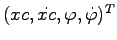, where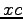is the position of the cart and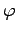is the pole angle.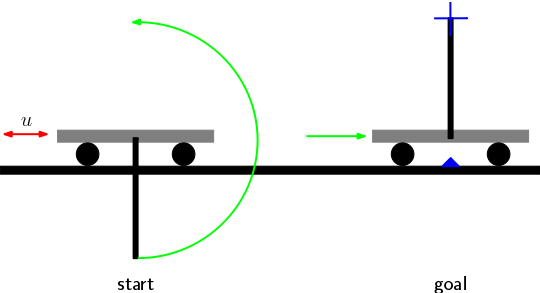a)
Download the MATLAB code for the cart-pole.2 The dynamics of the cart-pole for one time step is computed in the function cp_dyn.m. The stateof the cart-pole can be visualized with the function cp_vis.m (you don't have to change or call these two functions).

b)
Modify the file learn_cp.m that contains also the parameters for the cart-pole in the structure model (masses, length of the pole etc.) to implement an optimization algorithm that minimizes the error computed with the function cost_function.m.

c)
Modify the file cost_function.m that simulates the cart for a duration of 10 seconds and assigns an error value to the dynamics of the cart. You have to i) complete the function cost_function.m to implement a neural network controller that generates the horizontal force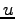that is applied to the cart at each time step, and ii) define an appropriate error function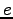(that scores the cart-dynamics for each time step), which has to be minimized.

d)
Neural network controller: Write the code to initialize and simulate a neural network without using the neural network toolbox. The input to the network for each time step should consist of the following 5 values: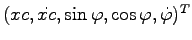. Choose an appropriate number of hidden neurons for the single hidden layer. The scalar output of the neural network, i.e. the force, should be bounded with values between -10 and 10.

Hints: Don't forget to optimize the bias values of the neurons. Use a tansig output neuron to obtained bounded output values.

e)
Error function: Use the state variables to construct an error functionthat outputs a value at each time step that assure that the pole remains in the upright positions after the upswing. Set the error to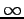if the cart leaves the interval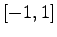. The total error (or cost) assigned to the cart movement could e.g. be the sum of allfor all time steps.

Hints: Take care of the fact that the values forreturned by the dynamical model in cp_dyn.m are not within the interval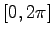.

f)
Analyze the best solution found and state the reasons for all choices you made in the MATLAB code.

Present your results clearly, structured and legible. Document them in such a way that anybody can reproduce them effortless.Next: Genetic Algorithm [3* P] Up: MLB_Exercises_2010 Previous: Comparison of Optimization Algorithms
Haeusler Stefan 2011-01-25